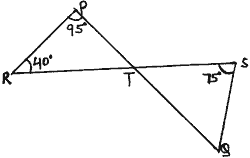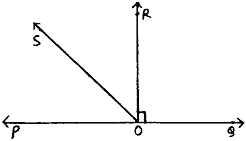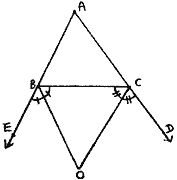Sunday , January 17 2021# 9th Mathematics Pre-Mid Exam Question Paper (2019-20)

## 9th CBSE Mathematics Pre-Mid Examination Question Paper (2019-20)

School Name: Himalayan Public School, Sector 13, Rohini, Delhi 110085 India
Time: 3 hr
M.M. 80 marks
Date: 24/06/2019
Class: IX
Subject: Mathematics

## General Introductions:

1. All questions are compulsory.
2. This question paper consists of 40 questions divided into five sections – A, B, C, D and 3-D DEXTER.
3. Section-A contains 16 questions of 1 mark each.
Section-B contains 3 questions of 2 mark each.
Section-C contains 8 questions of 3 mark each.
Section-D contains 6 questions of 4 mark each.
3-D DEXTER contains 4 questions of 1 mark each and 3 questions of 2 marks each.
4. Use of Calculators is not permitted.

## Section B: 9th Mathematics Pre-Mid Exam

#### Question: 19. In figure, lines PQ and RS intersect at a point T, such that ∠PRT = 40°, ∠RPT = 95° and ∠TSQ = 75°, find ∠SQT.## Section C: 9th Mathematics Pre-Mid Exam

#### Question: 24. Find:

1. mirror image of P(-1, 4) in x – axis.
2. perpendicular distance of Q(-2, -4) from x axis.
3. abscissa of the point R(3, 0).

#### Question: 25. In figure, AB || EF and CD || EF. Also EA ⊥ AB. If ∠BEF = 40°, then find x, y and z.#### Prove that ∠ROS = ½ (∠QOS – ∠POS).## Section D

#### Question: 32. In ΔABC, the side AB and AC of triangle are produced to points E and D respectively. If bisectrs BO and CO of ∠CBE and ∠BCD respectively meet at point O, then prove that ∠BOC = 90° – ½ ∠A.#### Question: 33. ABC is an equilateral triangle with side ‘a’.

1. Find the area of ΔABC using Heron’s formula.
2. If its perimeter is 180 cm, what will be the area of the triangle.

## 3-D DEXTER

#### Question: 40. Write shortcuts of the following: 

1. Rectangle tool
2. Offset tool
3. Rotate tool
4. Push Pull

## CBSE 9th Topics Removed for 2020-21

CBSE 9th Topics Removed for 2020-21: CBSE syllabus for all subjects of class 9 has …